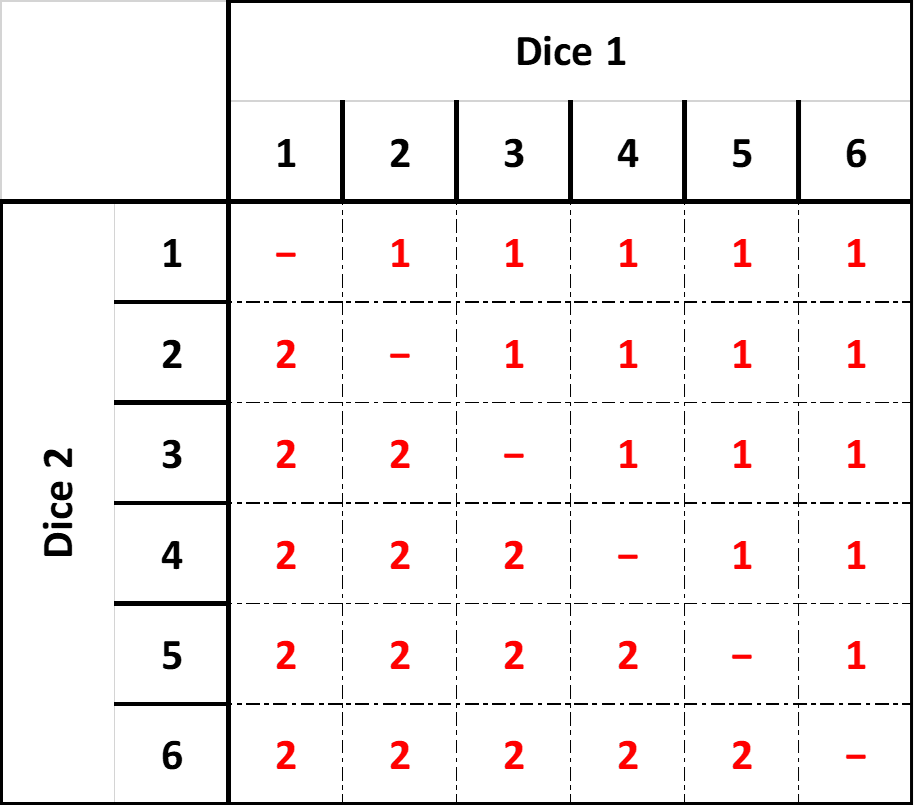# Dice duelling

In this activity we shall look at how the arrangement of numbers on a dice changes a person's probability of winning a dice-duel

## Basic dice duelling

To start let's consider a game where two players each roll a 6-sided die and the winner is whoever rolls the highest number. What is the probability of either opponent winning?

If you've already looked at the Investigating Dice Combinations activity, you will know that there are 36 different ways two standard 6-sided dice can be rolled. In this case instead of producing a matrix that illustrates totals, we can use the same idea to show which player wins.We can now visually observe that each player is just as likely to win as the other. The probability of a win for either player is 15 out of 36, as a simplified fraction this is written as 512 (here's a link to remind you how to simplify fractions). To write a percentage probability of winning we use 5 ÷ 12 × 100 = 41.67%

What is the probability of a draw?

Now let's say that if there is a draw, Player 1 automatically wins. If this was the case, what is the probability of Player 1 winning (in both a fraction and a percentage)?

Keeping the above rule that player one wins on a draw, add a new rule that player 2 gets to add one to their roll. So, if they both roll a 2 for example, player 2 wins because they get +1 to their roll. However, if player 1 rolls a 3 and player 2 rolls a 2, then it is a draw and player 1 wins. What are the new probabilities for both player 1 and player 2 winning? Remember to include the simplified fraction format and the percentage.

## Changing the dice

A normal 6-sided dice has sides labelled 1, 2, 3, 4, 5, 6. What is the mean average value of a dice roll? Here is a link to remind you how to calculate the mean average if you need it.

Let's now introduce some new 6-sided dice:

A red die with the sides labelled 1, 4, 4, 4, 4, 4.

A green die with the sides labelled 2, 2, 2, 5, 5, 5.

A blue die with the sides labelled 3, 3, 3, 3, 3, 6.

Which colour die do you think is the best for duelling? Why?

What is the mean average value of each of these dice? Does this change your mind in regards to which die is best for duelling? Why?

If you wish to experiment with this here is a link for a 6-sided dice template with blank sides for you to copy or print

For the next exercise you will need to create three matrices, like the one we used in the basic dice duelling, one for red vs blue, one for blue vs green and one for red vs green

What are the probabilites for each dice during these duels? Which dice beat which? Which colour was best?

## Rock, paper, dice....

These three dice acted in the same manner as the popular game of rock, paper, scissors. No one colour was better than all the others. This circular ordering of objects is called a non-transitive order.

## Extension

See what happens when you use 2 of each type of dice together. Do two red dice beat two blue dice, for example? Are they still non-transitive?

Up for more? Then how about mixing the colours of dice? Is there a combination of two dice that are better than the others?

## Answers

Once you've completed this activity, or if you are totally stuck, here are the answers with workings.